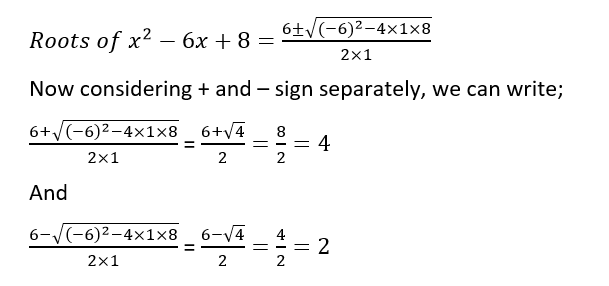Quadratic function is also a second-degree polynomial function. The graph of a quadratic function is a parabola. The parabola opens upwards if a graph is made for the quadratic formula.

The point at which the function attains maximum or minimum value is the vertex of the quadratic function. When we say second degree, then the variable is raised to the second power like

$$\begin{array}{l}x^{2}\end{array}$$
.

A quadratic function is of the form f(x) = ax2 + bx + c, where a, b, and c are the numbers with a not equal to zero.

The formula to solve a quadratic function is given by:

$\large x=\frac{-b\pm \sqrt{b^{2}-4ac}}{2a}$

Where,
a, b and c are the variables given in the equation.

This formula is used to solve any quadratic equation and get the values of the variable or the roots.

### Solved Example

Question: Solve:

$$\begin{array}{l}x^{2} – 6x + 8 = 0\end{array}$$

Solution

Given,

$$\begin{array}{l}x^{2} – 6x + 8 = 0\end{array}$$

Here,
= 1,=
b = -6
c = 8

Using the formula, to find the roots of equation, we have:

$$\begin{array}{l}x=\frac{-b\pm \sqrt{b^{2}-4ac}}{2a}\end{array}$$The roots are:

$$\begin{array}{l}x_{1}=4\end{array}$$
and
$$\begin{array}{l}x_{2}=2\end{array}$$

## Video Lesson